# Simple Imperfect Squared Squares (SISSs) ; Orders 13 to 32

### Imperfect Squared Squares

Imperfect squared squares are more numerous (at a given order) than perfect squared squares. The counts of SISS by order are listed in the OEIS as sequence A002962 ; The number of simple imperfect squared squares of order n.  Counts were obtained by processing all 3-connected planar graphs up to 30 edges as resistor networks subject to an electromotive force in each edge to obtain square tilings.  All rectangles, perfect squared squares and compound imperfect squared squares have been filtered out, and any remaining duplicates eliminated, to arrive at these counts.

#### The number of Simple Imperfect Squared Squares (SISSs) by Order, in bouwkampcodes & pdf (orders 13 - 24 only) & postscript (orders 25 -> 31 only);

SISSs are by definition, imperfect, (have at least 2 squares the same size), however it is possible to get perfect squares from imperfect squares. One can 'derive' SPSSs (Simple Perfect Squared Squares) of order n-2 from SISSs of order n. This provides a means of verifying the SPSS counts obtained in lower orders.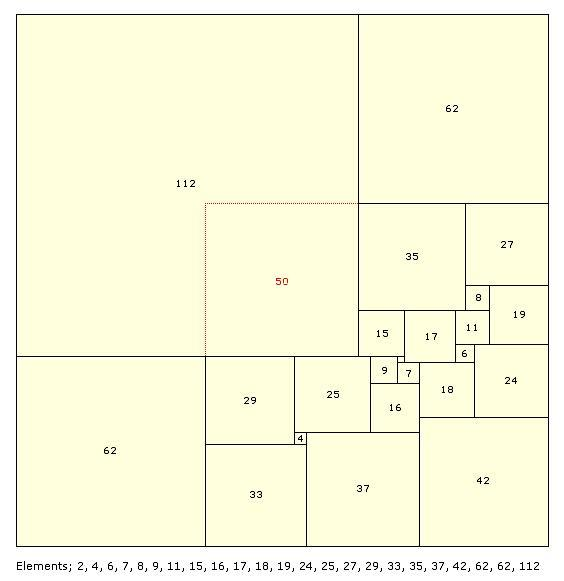Order 23: 174 x 174 SISS with Duijvestijn's order 21 SPSS derived. The two 62 squares and the 112 square are removed and replaced with a square that is the difference of the two sizes, 112 - 62 = 50.

## Imperfection can result in symmetry

As a result of squares in the tiling being the same size, some of these 'imperfect' tilings have interesting symmetrical arrangements. There are dissections which invariant under a 90 degree rotation, 180 degree rotation, reflection about a diagonal and reflection about centre axes. A collection of symmetrical SISSs is here!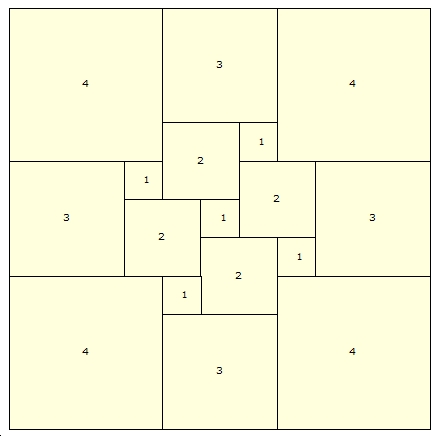SISS (Simple Imperfect Squared Square), Order 17: 11 x 11, the tling is invariant under 90 degree rotations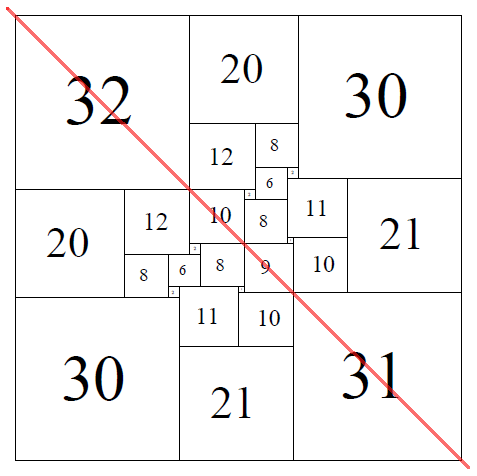SISS (Simple Imperfect Squared Square), Order 28: 82 x 82, the tiling is symmetrical about the diagonal axis.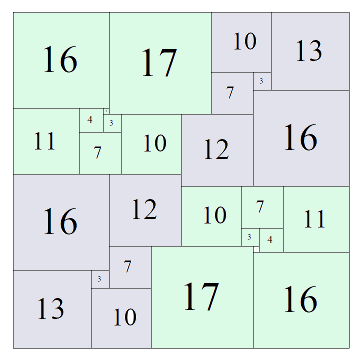SISS (Simple Imperfect Squared Square), Order 28: 28 x 28, rotational symmetry, the tiling is invariant under 180 degree rotations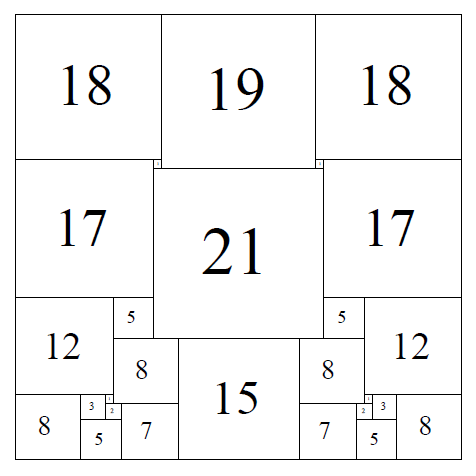SISS (Simple Imperfect Squared Square), Order 27: 55 x 55, the tiling is symmetrical about the vertical axis